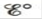# Sketch the galvanic cells based on the following half-reactions. Show the direction of electron flow, show the direction of ion migration through the salt bridge, and identify the cathode and anode. Give the overall balanced equation, and determine for the galvanic cells. Assume that all concentrations are 1.0 M and that all partial pressures are 1.0 atm. a. b.### Chemistry: An Atoms First Approach

2nd Edition
Steven S. Zumdahl + 1 other
Publisher: Cengage Learning
ISBN: 9781305079243

#### Solutions

Chapter
Section### Chemistry: An Atoms First Approach

2nd Edition
Steven S. Zumdahl + 1 other
Publisher: Cengage Learning
ISBN: 9781305079243
Chapter 17, Problem 42E
Textbook Problem
1 views

## Sketch the galvanic cells based on the following half-reactions. Show the direction of electron flow, show the direction of ion migration through the salt bridge, and identify the cathode and anode. Give the overall balanced equation, and determinefor the galvanic cells. Assume that all concentrations are 1.0 M and that all partial pressures are 1.0 atm.a.b.(a)

Interpretation Introduction

Interpretation:

The reaction taking place in two different galvanic cell is given. The sketch of the given galvanic cell along with the cathode and anode and the direction of electron flow, direction of flow of migration of ions through salt bridge, the balanced chemical equation and calculation of E° is to be stated.

Concept introduction:

The galvanic cell converts chemical energy into electrical energy while the electrolytic cell converts electrical energy into chemical energy.

The species at anode undergoes oxidation while the species at cathode undergoes reduction and the electrons generated at the anode are transferred through wire to the cathode.

To determine: The sketch of the cell given in the chemical equation; the direction of electron flow, identification of the cathode and anode and the migration of ions from salt bridge; the balanced chemical equation for given reaction and the value of overall cell potential.

### Explanation of Solution

The galvanic cell based on the given reaction is represented by the following sketch.

The anode compartment has platinum electrode in contact with O2 and the cathode compartment has platinum electrode in contact with H2O2 .

The galvanic cell is represented as,

Figure 1

The electrons flow from the anode compartment having platinum electrode in contact with O2 to the cathode compartment having platinum electrode in contact with H2O2 . The cations flow towards cathode while anions flow towards anode via salt bridge.

The species at anode undergoes oxidation while the species at cathode undergoes reduction reaction. Therefore, the electrons generated at anode travel to cathode through wire in an electrical circuit. Inside the solution the flow of ions occur so as to maintain the overall charge of the reaction.

The overall balanced chemical equation for given reaction (a) is,

2H2O22H2O+O2

The reaction taking place at cathode is,

H2O2+2H++2e2H2OE°red=1.78V

The reaction taking place at anode is,

H2O2O2+2H++2eE°ox=0.68V

The final equation is,

2H2O22H2O+O2

The value of E°cell is calculated as 1

(b)

Interpretation Introduction

Interpretation:

The reaction taking place in two different galvanic cell is given. The sketch of the given galvanic cell along with the cathode and anode and the direction of electron flow, direction of flow of migration of ions through salt bridge, the balanced chemical equation and calculation of E° is to be stated.

Concept introduction:

The galvanic cell converts chemical energy into electrical energy while the electrolytic cell converts electrical energy into chemical energy.

The species at anode undergoes oxidation while the species at cathode undergoes reduction and the electrons generated at the anode are transferred through wire to the cathode.

To determine: The sketch of the cell given in the chemical equation; the direction of electron flow, identification of the cathode and anode and the migration of ions from salt bridge; the balanced chemical equation for given reaction and the value of overall cell potential.

### Still sussing out bartleby?

Check out a sample textbook solution.

See a sample solution

#### The Solution to Your Study Problems

Bartleby provides explanations to thousands of textbook problems written by our experts, many with advanced degrees!

Get Started

Find more solutions based on key concepts
Two tablespoons of peanut butter offer about the same amount of protein as a hot dog. T F

Nutrition: Concepts and Controversies - Standalone book (MindTap Course List)

Why are titanium oxide features visible in the spectra of only the coolest stars?

Horizons: Exploring the Universe (MindTap Course List)

What is the normality of the base in Question 101?

Introductory Chemistry: An Active Learning Approach

What characteristics are unique to marine mammals?

Oceanography: An Invitation To Marine Science, Loose-leaf Versin

Review. A light balloon filled with helium of density 0.179 kg/m3 is tied to a light string of length L = 3.00 ...

Physics for Scientists and Engineers, Technology Update (No access codes included)